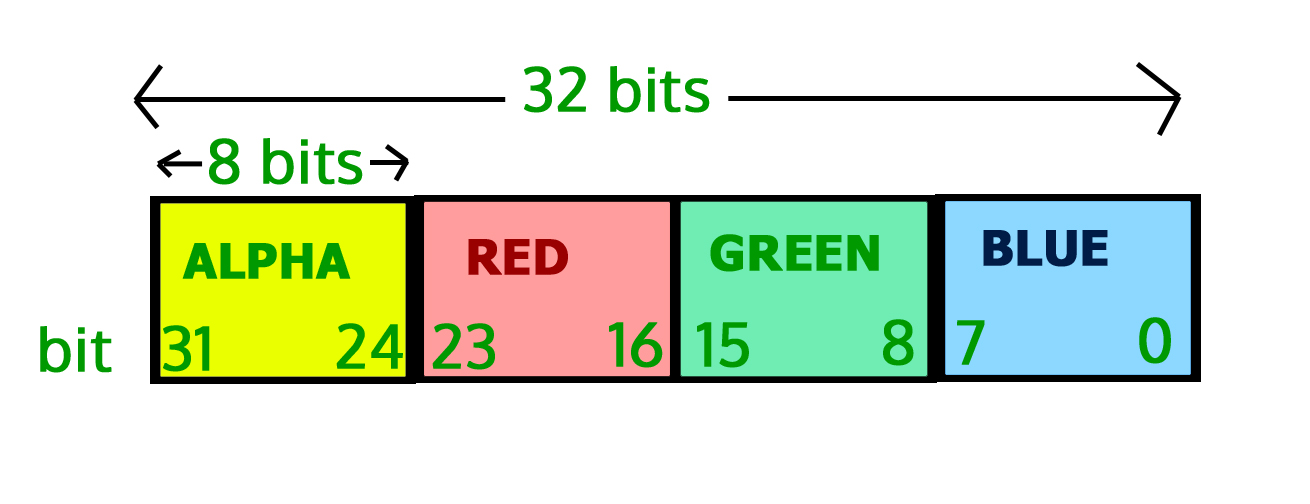Related Articles

# Image Processing In Java | Set 2 (Get and set Pixels)

• Difficulty Level : Hard
• Last Updated : 30 Jun, 2021

We strongly recommend to refer below post as a prerequisite of this.
Image Processing in Java | Set 1 (Read and Write)
In this set, we will learn about pixels of image. How we can get pixel values of image and how to set pixel values in an image using Java programming language.
Pixels are the smallest unit of an image which consists of four components Alpha (transparency measure), Red, Green, Blue and in short (ARGB).
The value of all the components lie between 0 and 255 both inclusive. Zero means the component is absent and 255 means the component is fully present.
Note:
Since, 28 = 256 and the value of the pixel components lie between 0 and 255, so we need only 8-bits to store the values.
SO, total number of bits required to store the ARGB values is 8*4=32 bits or 4 bytes.
As the order signifies Alpha acquires leftmost 8 bits, Blue acquires rightmost 8 bits.
Thus the bit position :
For blue component being 7-0,
For green component being 15-8,
For red component being 23-16,
For alpha component being 31-24,
Pictorial representation of indexes:## Java

 `// Java program to demonstrate get and set pixel``// values of an image``import` `java.io.File;``import` `java.io.IOException;``import` `java.awt.image.BufferedImage;``import` `javax.imageio.ImageIO;` `public` `class` `GetSetPixels``{``    ``public` `static` `void` `main(String args[])``throws` `IOException``    ``{``        ``BufferedImage img = ``null``;``        ``File f = ``null``;` `        ``//read image``        ``try``        ``{``            ``f = ``new` `File(``"G:\\Inp.jpg"``);``            ``img = ImageIO.read(f);``        ``}``        ``catch``(IOException e)``        ``{``            ``System.out.println(e);``        ``}` `        ``//get image width and height``        ``int` `width = img.getWidth();``        ``int` `height = img.getHeight();` `        ``/*  Since, Inp.jpg is a single pixel image so, we``            ``will not be using the width and height variable */` `        ``/* get pixel value (the arguments in the getRGB method``         ``denotes the  coordinates of the image from which the``         ``pixel values need to be extracted) */``        ``int` `p = img.getRGB(``0``,``0``);` `        ``/* We, have seen that the components of pixel occupy``           ``8 bits. To get the bits we have to first right shift``           ``the 32 bits of the pixels by bit position(such as 24``           ``in case of alpha) and then bitwise ADD it with 0xFF.``           ``0xFF is the hexadecimal representation of the decimal``           ``value 255.  */` `        ``// get alpha``        ``int` `a = (p>>``24``) & ``0xff``;` `        ``// get red``        ``int` `r = (p>>``16``) & ``0xff``;` `        ``// get green``        ``int` `g = (p>>``8``) & ``0xff``;` `        ``// get blue``        ``int` `a = p & ``0xff``;` `        ``/*``        ``for simplicity we will set the ARGB``        ``value to 255, 100, 150 and 200 respectively.``        ``*/``        ``a = ``255``;``        ``r = ``100``;``        ``g = ``150``;``        ``b = ``200``;` `        ``//set the pixel value``        ``p = (a<<``24``) | (r<<``16``) | (g<<``8``) | b;``        ``img.setRGB(``0``, ``0``, p);` `        ``//write image``        ``try``        ``{``            ``f = ``new` `File(``"G:\\Out.jpg"``);``            ``ImageIO.write(img, ``"jpg"``, f);``        ``}``        ``catch``(IOException e)``        ``{``            ``System.out.println(e);``        ``}``    ``}``}`

Note : This code will not run on online IDE as it needs an image on disk.
In the next set we will be learning how to convert a colored image to grayscale image in JAVA.
This article is contributed by Pratik Agarwal. If you like GeeksforGeeks and would like to contribute, you can also write an article using write.geeksforgeeks.org or mail your article to review-team@geeksforgeeks.org. See your article appearing on the GeeksforGeeks main page and help other Geeks.